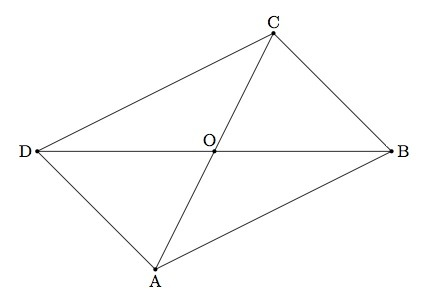# Is this a parallelogram?

Alignments to Content Standards: G-CO.C.11

In quadrilateral $ABCD$ pictured below, $\overline{AB}$ is congruent to $\overline{CD}$ and $\overline{BC}$ is congruent to $\overline{AD}$.From the given information, can we deduce that ABCD is a parallelogram? Explain.

## IM Commentary

This task develops an alternative characterization of a parallelogram in terms of congruence of opposite sides. The reverse implication is also true: the opposite sides of a parallelogram are congruent so a quadrilateral is a parallelogram if and only if its opposite sides are congruent. The first argument given here uses congruent triangles and so the student needs to draw the two diagonals of $ABCD$. If students do not think of this technique, the teacher may wish to provide some guidance.

A second argument builds upon the construction of the first solution but concludes that $ABCD$ is a parallelogram using a rigid motion argument instead of congruence of alternate interior angles. The key points in this argument are that a 180 degree rotation maps $ABCD$ to itself and that the image of a line $\ell$ by a 180 rotation is parallel to $\ell$. This second solution is mostly appropriate if the students are studying transformations of the plane and symmetries of geometric figures. The teacher may need to provide some assistance or prompting in this direction.

## Solutions

Solution: 1 Constructing transverses for the parallel sides

Quadrilateral $ABCD$ is a parallelogram if $\overleftrightarrow{AB}$ is parallel to $\overleftrightarrow{DC}$ and $\overleftrightarrow{AD}$ is parallel to $\overleftrightarrow{BC}$. Below is a picture of $ABCD$ with the diagonal $\overline{BD}$ drawn:We know that $\overline{AB}$ is congruent to $\overline{CD}$ and $\overline{AD}$ is congruent to $\overline{BC}$ by hypothesis. Since $\overline{BD}$ is congruent to itself, $\triangle ABD$ is congruent to $\triangle CDB$ by SSS. This means that $\angle ABD$ and $\angle CDB$ are congruent. Since $\overline{DB}$ is a transverse for $\overleftrightarrow{DC}$ and $\overleftrightarrow{AB}$ this means that $\overleftrightarrow{DC}$ and $\overleftrightarrow{AB}$ are parallel.

Using the diagonal $\overline{AC}$, the same argument can be applied to show that $\triangle DCA$ is congruent to $\triangle BAC$. This means that $\angle ACB$ and $\angle CAD$ are congruent from which we conclude that $\overleftrightarrow{AD}$ and $\overleftrightarrow{BC}$ are parallel. Since the two pair of opposite sides of $ABCD$ are contained in parallel lines, $ABCD$ is a parallelogram.

Solution: 2 Triangle congruence and rotation

Below is a picture of $ABCD$ with both diagonals drawn and their point of intersection labelled:By hypothesis $\overline{AB}$ is congruent to $\overline{CD}$ and $\overline{AD}$ is congruent to $\overline{BC}$. Since $\overline{AC}$ is congruent to itself, we can apply SSS to conclude that $\triangle ABD$ is congruent to $\triangle CDB$. Since they are corresponding parts of congruent triangles $\angle ABD$ is congruent to $\angle CDB$ Thus $\angle ABO$ is congruent to $\angle CDO$.

The vertical angles $COD$ and $AOB$ are also congruent and so the AA similarity theorem says that $\triangle COD$ is similar to $\triangle AOB$. But $\overline{CD}$ is congruent to $\overline{AB}$ so the scale factor between triangles $AOB$ and $COD$ is one and they are congruent. So $\overline{AO}$ is congruent to $\overline{CO}$ and $\overline{BO}$ is congruent to $\overline{DO}$. This means that $O$ is the midpoint of $\overline{AC}$ and of $\overline{BD}$. So rotation by 180 about $O$ switches $A$ and $C$ and also switches $D$ and $B$. Hence rotation by 180 degrees maps $ABCD$ to itself and this means that $ABCD$ is a parallelogram.